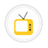you are here->home->Biotechnology and Biomedical Engineering->Systems Biology Virtual Lab
Systems Biology Virtual Lab

This virtual lab consist of modelling and simulation experiments for UG/PG students in bioinformatics and computational biology to understand biological processes using a systems biology approach.Mathematical modeling and simulating of Biochemical networkTo learn how to develop and simulate a mathematical modelImport and simulate models from different databases To learn how to import, model and simulate the model using databasesTo Import and simulate a model from the repository Import and simulate the model from the repository and understand the functions of genes based on their evolutionary relationships.SBML-A markup language for mathematical models in systems biology using cell designerSystems Biology Markup Language (SBML) is a xml (eXtensible Markup Language) based language. It is a description language for simulations in systems biology. SBML is suitable for representing biochemical networks, which includes cell signaling pathwaysCreating and Visualizing a Simple Network ModelTo create and visualize a simple network using system biology visualization software'sAnalysis of biological networks for feature detectionTo Analyse the different features for better understanding of interactions in the networkIntegrating Biological Networks and Microarray Expression dataTo learn the integration of expression data with biological network and analyse the networkAnalyzing the network by finding sub modules To learn how to analyze the network by finding the sub modules based on the expression dataCopyright @ 2023 Under the NME ICT initiative of MHRD